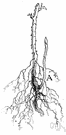# root system

(redirected from Dynkin diagram)
Also found in: Thesaurus, Medical.

## root system

n.
1. All of the roots of a plant.
2. The arrangement of the roots of a plant: a branched root system.
ThesaurusAntonymsRelated WordsSynonymsLegend:
 Noun 1root system - a developed system of roots    rootagesystem, scheme - a group of independent but interrelated elements comprising a unified whole; "a vast system of production and distribution and consumption keep the country going"root - (botany) the usually underground organ that lacks buds or leaves or nodes; absorbs water and mineral salts; usually it anchors the plant to the ground
Based on WordNet 3.0, Farlex clipart collection. © 2003-2012 Princeton University, Farlex Inc.
References in periodicals archive ?
In case of [D.sub.4] the group of symmetry of the Dynkin diagram G' is isomorphic to the symmetric group [S.sub.3] acting on the set of three vertices (1,3,4} of the Dynkin diagram via their permutations.
The main result of this paper is the determination of the Lie algebra [n.sub.q] when [theta] = 2 and the generalized Dynkin diagram of q is connected.
They are also well-understood in the context of cluster algebras, where they are just one among several Cambrian lattices of type A, depending on the choice of an orientation of the Dynkin diagram, see Reading (2006); Reading and Speyer (2009).
It is the Dynkin diagram of the given group equipped with certain action of the absolute Galois group.
Let [delta] be an ADE Dynkin diagram with vertex set I.
Concluding Remarks 6.1 It follows from Lemma 3 and the results obtained recently in [3,4] that for any connected positive (resp., principal) poset J, there exists a simply laced Dynkin diagram DJ [member of] {[A.sub.m], [D.sub.m], [E.sub.6], [E.sub.7], [E.sub.8]} (resp., a simply laced Euclidean diagram DJ), uniquely determined by J, such that the symmetric Gram matrices [G.sub.J], [G.sub.DJ] are Z-congruent.
Let X be a Dynkin diagram of finite type, and A = ([a.sub.ij]) be the corresponding Cartan matrix.
Definition 3.3 A subset of simple roots K [subset or equal to] [DELTA] is called connected if the induced Dynkin diagram of K is a connected subgraph of the Dynkin diagram of [DELTA].
The Dynkin diagram r associated to (W, S) is the graph with vertex set S and, for each pair s, t with [m.sub.st] [greater than or equal to] 3, an edge between s and t labeled by [m.sub.st] (when [m.sub.st] = 3 the edge is left unlabeled).
The parabolic subgroup is associated to roots 2 and 4 of the Dynkin diagram of [A.sub.4].
There is a natural dihedral symmetry of the Dynkin diagram of affine type A.
If the rank of g is sufficiently large, X does not depend on g itself, but only on the attachment of the affine Dynkin node 0 to the rest of the Dynkin diagram. See Table 1.

Site: Follow: Share:
Open / Close